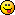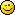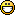#### Topic: Conditional insertion

I would like to insert some expression depending if another expression is empty or not.  It could be useful to add such possibilityMy case is the following:

If %Ev is not empty, then insert it rounded by dot-parenthesis *.(%Ev).*,
ElseIf %EV is not empty then insert it rounded by dot-squared brackets *.[%EV].*,
Else insert nothing *.*.

This could be something like:
%Ev?(x,y) => if %Ev is empty or zero, then insert x else insert y
or more sophisticated:
%Ev=a?(x,y) => with "=" is an comparison operator (= or ==, <=, <> ou !=,...)
or another syntax.

Great application!
Michel.

#### Re: Conditional insertion

Bonjour Michel,

Even if it is not obviousyou already can do this.
Here is what the expression could be:
%b.(%Ev)..[%EV]..*;%b(s/(\.\(.+\)\.)\.\[.*\]\./\1/)(".().")(".[].").*

It is a combination of sub-expressions and string modifiers.
Of course it would be much easier to use a specific syntax like the one you suggest.

For sure the expression grammar has to be improved. I'll have to work on this.
Any contribution is welcome.

Tiens, il n'est jamais trop tard pour bien faire : Merci pour ta réponse